# Product of ideals

## Definition

### Definition with symbols

Suppose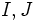$I,J$ are ideals in a commutative unital ring$R$. Then the product of ideals$I$ and$J$, denoted$IJ$, is defined in the following equivalent ways:

• It is the additive subgroup generated by all elements of the form$ab$ where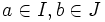$a \in I, b \in J$
• It is the smallest ideal containing all elements of the form$ab$ where$a \in I, b \in J$
• It is the ideal defined as the set of elements of the form:$\sum_{i=1}^n a_ib_i$

with$a_i \in I, b_i \in J$

## Facts

• Product of ideals is commutative and associative. Hence, we can talk of the product of more than two ideals by simply writing them as a string. The product of ideals$I_1, I_2, \ldots, I_n$, denoted$I_1I_2\ldots I_n$, is the subgroup generated by elements of the form$a_1a_2\ldots a_n$ where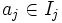$a_j \in I_j$ for every$j$
• We can also use this to define the notion of power of an ideal. For an ideal$I$, the ideal$I^n$ is simply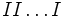$II \ldots I$ written$n$ times. It is the ideal generated by$n$-fold products of elements from$I$, and need not be the same as the ideal generated by$n^{th}$ powers of elements from$I$
• In general, the set of products of elements from$I$ and$J$ is not additively closed. An important exception is the situation where either$I$ or$J$ is a principal ideal.
• The product of two ideals is contained in their intersection, and contains the square of their intersection. In symbols: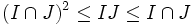$(I \cap J)^2 \le IJ \le I \cap J$